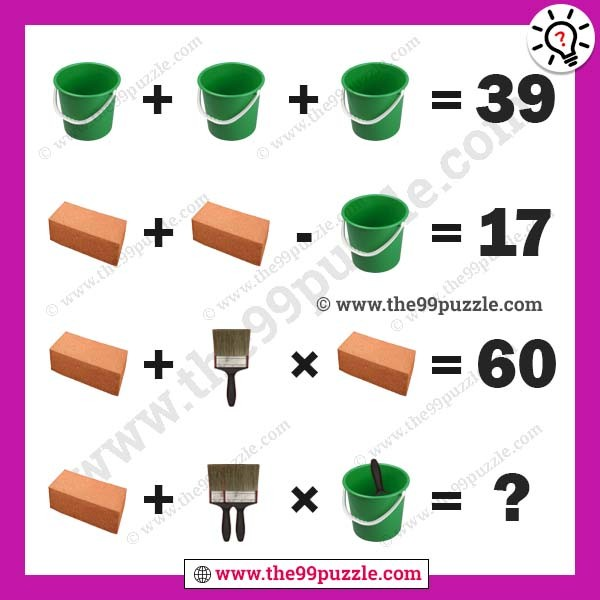# Bucket, bricks, brush math puzzle with answer – Puzz193

Bucket, bricks, brush math puzzle for school students. This picture puzzle question will test your logical skills. Solve these equations to find the value of the Bucket, bricks, and brush. Can you solve the last equation to find the value of the picture puzzle?###### Explanation:

Bucket + Bucket + Bucket = 39

Bricks + Bricks − Bucket  = 17

Bricks + Brush × Bricks = 60

Bricks + (2×Brush) × (Bucket+Brush) = ?

Bucket = 13

Bricks = 15

Brush = 3

13 + 13 + 13 = 39

15 + 15 − 13  = 17

15 + 3 × 15 = 60

15 + (2×3) × (13+3) = 111